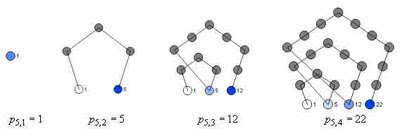## Thursday, July 31, 2008

### Digit Patterns in Power Sequences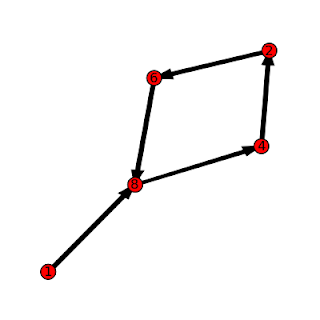Looking at the last few digits that appear in the numbers that form the sequence b^0, b^1, b^2, b^3, ... for b a positive integer, you'll notice that the digits will always begin to repeat after a certain point. For example, looking at the last digit of the sequences for b = 2, 3, and 4 we have the sequences

b = 2: 1, 2, 4, 8, 6, 2, 4, 8, 6, ...
b = 3: 1, 3, 9, 7, 1, 3, 9, 7, ...
b = 4: 1, 4, 6, 4, 6, 4, 6, ...

If we look at the sequence of last two digits of these sequence where b =2 we have

b = 2: 1, 2, 4, 8, 16, 32, 64, 28, 56, 12, 24, 48, 96, 92, 84, 68, 36, 72, 44, 88, 76, 52, 4, ...

This sequence then repeats the loop that began at 4.

We can describe these sequences as T_b,d(n) = (b^n)mod 10^d. Recursively, T_b,d(n) = (T_b,d(n-1)*b)mod 10^d

These sequences are always eventually periodic. Although these sequences are simple to understand and calculate, there are several interesting ways of describing them.

For example, you can think of the elements of T_b,d as a commutative monoid, with multiplication defined as a*b = (a*b)mod 10^d. They form a monoid since 1 is always a member, and you can show that T_b,d is closed under the * operation. It turns out that for some values of b, and d, T_b,d is a group.

You can also think of this set as a finite state machine or graph, where each element is a node and the transition from one node to the next is defined by the operation *b mod 10^d. This provides a nice way of displaying the sequences. The pictures in this post were created by writing a short program to calculate the sequences, and then formatting the output to draw a di-graph in SAGE. The graph at the top of the post is for b=8, d=1, while the graph below is for b=2, d=2. The graph at the bottom of the page is for b=7, d=1.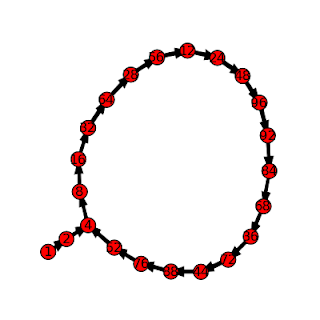## Tuesday, July 22, 2008

### Higher Polygonal Numbers and Pascal's TriangleThe third diagonal column in Pascal's Triangle (r = 2 in the usual way of labeling and numbering) consists of the triangular numbers (1, 3, 6, 10, ...) - numbers that can be arranged in 2-dimensional triangular patterns. The fourth column of Pascal's triangle gives us triangular-based pyramidal numbers (1, 4, 10, 20, ...), built by stacking the triangular numbers. The columns further out give "higher dimensional" triangular numbers that arise from stacking the triangular numbers from the previous dimension.

It is not by coincidence that the triangular and higher-dimensional triangular numbers appear in Pascal's Triangle. If you think about layering of polygonal numbers in terms of equations, you get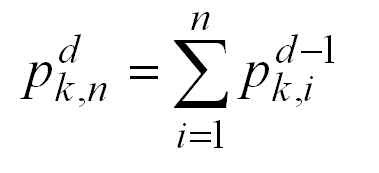In the above equation p^d_(k,n) is the nth k-polygonal number of dimension d. Triangular numbers are the 3-polygonal numbers of dimension 2, square numbers are the 4-polygonal numbers of dimension 2, "square based pyramidal numbers" would be denoted as p^3_(4,n).

From the sum above, you can obtain this equation:Which looks very much like the Pascal Identity C(n,r) = C(n-1,r-1) + C(n-1,r), except for some translation of the variables. To be precise, if we consider the case where k=3 and use r = d and n' = n+d-1 we can translate the triangular numbers into the appropriate positions in Pascal's Triangle.

Along with the definitions for the end columns, the Pascal Identity allows us to generate the whole triangle. This suggests the following strategy for calculating the higher k-Polygonal numbers: create a modified Pascal's Triangle whose first column is equal to k-2 (instead of 1), and whose last column is equal to 1 (as usual). This modified Pascal's Triangle is generated using these initial values and the usual Pascal Identity.

Here is an example with k=5, which sets the first column values equal to 3 (except for the top value, which we keep as 1) and yields the pentagonal numbers (column 3) and the higher pentagonal numbers.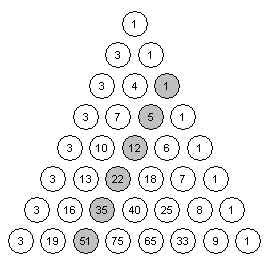The formula for these modified Pascal Triangles is given by this equation: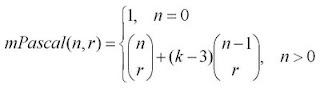If we apply the change of variables mentioned above, we can obtain this general formula for the higher polygonal numbers in terms of combinations: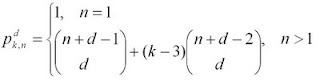This formula illustrates how polygonal numbers are built out of triangular numbers. It says that the nth d-dimensional k-polygonal number is equal to the nth d-dimensional triangular number, plus (k-3) copies of the n-1 d-dimensional triangular number. This is a little easier to understand when you forget about the higher-dimensions and look at the regular 2-dimensional polygonal numbers, as described in another post.

## Thursday, July 17, 2008

### Pi Color Map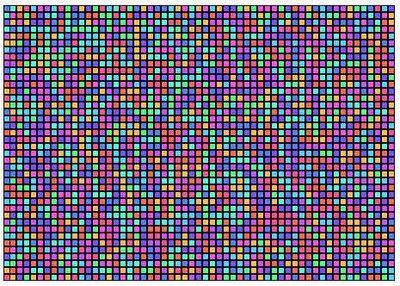John Sims has created a number of pi-related art works. One, the Pi Color Map, can be recreated effectively using TinkerPlots. The image above is one such Pi Color Map, using 2281 digits of pi.

Here are some instructions for creating a Pi Color Map in Tinkerplots.

1. Obtain a listing of the digits of pi - up to a reasonable number. You can get the digits from several sites, including the pi day site.

2. Paste your listing to a text document, and get them arranged into a single column. One strategy for doing this is and use the find/replace feature of a word-processor to replace each number with the number itself plus a line-break(e.g. in Word, replace 2 with 2^l, etc.).

3. If you've included the decimal point, remove it. For the first line of your document, provide a heading like pi_expansion. This will be your TinkerPlots attribute.

3. Import the text file into TinkerPlots using the File>Import menu.

4. Create a new attribute called digit whose formula is digit=concat("",pi_expansion). This creates a categorical data type that TinkerPlots won't treat numerically. This is what you will use as your color key. Using the pi_expansion attribute gives a spectrum of color, rather than distinct colors for each number.

5. Create a new attribute called place, whose formula is place=caseIndex. This is what you will order your plot by.

6. Create a new plot, lock the color key on the digit attribute. Select the place attribute and press the Order By button.

7. Change your icon type to small squares, and stack the cases.

You can play with different options to get different effects for your color map.

One nice thing about doing this in TinkerPlots is that you can investigate the data further. The color map plot highlights the apparent randomness of the pi expansion, but you can also create other attributes and plots to investigate things like the running average of the digits, occurrences of consecutive digits, and the overall distribution of the digits (it should be uniform).

If you are having trouble creating this yourself, a Tinkerplots file for this is here, or you can use the data file here.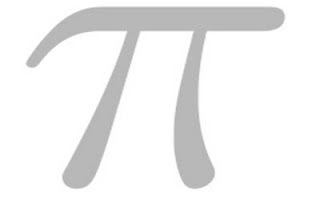Postscript: here is another look at the same picture of pi.

### Digit Patterns in Square Numbers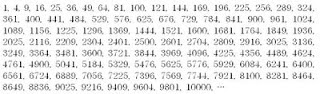If you take a look at the square numbers (n^2, n a positive integer), you'll notice plenty of patterns in the digits. For example, if you look at just the last digit of each square, you'll observe the repeating pattern 1, 4, 9, 6, 5, 6, 9, 4, 1, 0, ... If you construct a graph of "last digit" vs n (like the one below, built with Fathom), the symmetry and period of this digit pattern is apparent.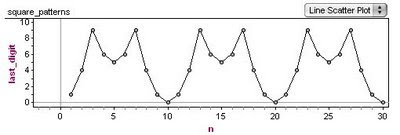Why does this happen? The periodic nature of the pattern is easy to understand - when you square a number, only the digit in the ones place contributes to ones place of the product. For example, 22*22 and 32*32 are both going to have a 4 as their last digit - the values in the tens place (or any other place other than the ones) do not affect what ends up as the last digit.

The reason for the symmetry about n=5 is a little less obvious. To see what is going on, it is helpful to use modular arithmetic and to realize that " last digit of n" is the same as "n mod 10". Considering what 10-n looks like mod 10 after it is squared, we have the equation below.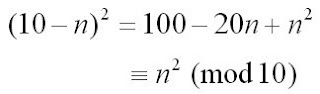This tells us that the last digit of (10-n)^2 is the same as the last digit of n^2, because everything else that is different about these two numbers is divisible by 10.

If you look at the last two digits of the square numbers, you see another repeating pattern that has similar symmetries.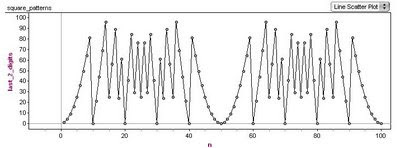This is a nice looking graph - the period is 50 with a line of symmetry at n=25. You can think about it in the same way as the one-digit case, this time the symmetry is understood by looking at (50-n)^2 mod 100. (Looking at numbers mod 100 tells us their last two digits.)

If you decide to investigate patterns in cubes or higher powers, you'll see somewhat similar results. Using the binomial theorem and modular arithmetic, you can see why even powers give symmetry similar to the n^2 case, while odd powers do not (although all are periodic).

This graph shows the pattern in the last digit of n^3.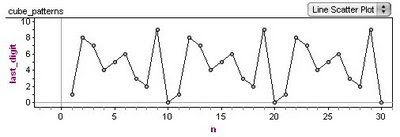This last graph shows the pattern for the last two digits of n^4.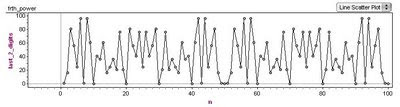## Thursday, July 10, 2008

### Knot tiles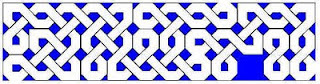Knot patterns (which might not actually represent knots, but just braids or plaits), like the one shown here, are common in Celtic design.

One way to create knot patterns is to use a set of tiles that are put together following a few basic rules. This is not, as far as I know, a standard way to create these patterns - the book by Aidan Meehan, provides a technique for drawing the patterns by hand, rather than laying them out as tiles.

A wide range of knot patterns, including the one shown above, can be created using the set of six tiles shown below. These were constructed using Geometer's Sketchpad.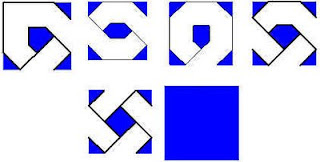The rules for putting these together are reasonably clear, but can be formalized. You can even come up with a notation for the tiles and formal rules for assembling them into knot patterns.

When laying down the tiles, you have the option of rotating them - some of the tiles don't alter with rotation, some of them can be placed in two ways, some in four.

A nice property of this set is that you can't paint yourself into a corner when using it - you can always find a tile that will compose with the pattern that you have started. This property means that this set of tiles is closed, in a certain sense.

A wider range of patterns can be created if you add tiles like the ones below to your set.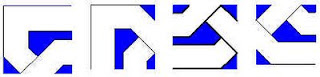Unfortunately, when you include these tiles, your set is no longer closed. An open question (at least as far as I know) is - how many more tiles would you need to add in order to create a closed set of tiles that includes these? Also, what would these tiles look like? Do they lead to "reasonable" looking knots?

The knot pattern below (better described as a woven set of three links) was made using tiles including the ones from the "extended" set above.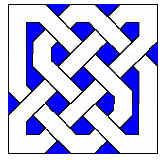## Wednesday, July 9, 2008

### Sonobe cut-out-module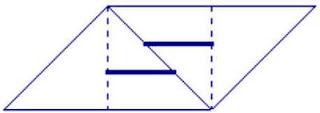Although creating origami sonobe units is likely to be considered by most to be an essential part of making sonobe polyhedra, a cut-out version of the sonobe module can be used to help beginners learn how to weave the units together.

The unit pictured here can be reproduced multiple times in a document (a PowerPoint slide works well, since you don't have to worry about margins), and printed onto card stock. Printing onto card stock gives a solid unit to work with, while regular paper will likely be too flimsy.

After printing, cut out each unit and cut slits into them along the bold horizontal lines (a utility blade or exacto-knife works well). Depending on whether you want to hide the printing or not, you can mountain-fold the diagonal line and vally-fold the dotted vertical lines, or vice-versa (all modules should be folded the same).

You will need 6 units for a cube, 12 for an "augmented octahedron", and 30 for an augmented icosahedron. Models can also be assembled from 3 units (a triangular di-pyramid) 9 units (two fused cubes), and other combinations.

The main idea in creating this module was to create a set of reusable units that could be used in professional development workshops for teachers learning modular origami for the first time. After seeing how the units hold together, the next step is to learn how to fold the units from paper.

Instructions for assembling the units are available here, among other places. To construct smaller modules, simply connect less than 5 units around. A 4 unit base will form the pattern for the augmented octahedron (which will take a total of 12 units). A 3 unit base will form the pattern for the cube (which will take a total of 6 units).

## Tuesday, July 8, 2008

### Drawing Polygonal Numbers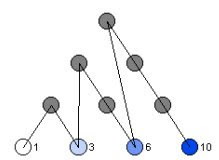The diagram above (known as the tetractys) shows the first four triangular numbers (1, 3, 6, 10, ...). Although there is a simple formula for calculating these numbers directly, t(n) = 1/2(n(n+1)), constructing them by these layered-triangle diagrams helps to show their geometric and recursive properties.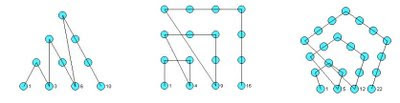More generally, polygonal numbers arise from counting arrangements of dots in regular polygonal patterns. Larger polygons are built from smaller ones of the same type by adding additional layers of dots, called gnomons. Beginning with a single dot, k-sided polygons are built by adding gnomons consisting of k-2 segments, with each segment of the gnomon having one more dot than the segments of the previous layer. In this way, the nth gnomon consists of segments each n dots long, but with k-3 dots shared by adjoining segments (the corners).

This post describes how you can draw figures that illustrate the polygonal numbers and explore the polygonal numbers in general (triangular, square, pentagonal, hexagonal, etc.) using either or Fathom. Both TinkerPlots and Fathom work well, but TinkerPlots creates nicer pictures, and allows for captions directly on the graph.

Without describing the details of how you create Fathom or TinkerPlot documents, here are the attributes that you will want to define in order to draw diagrams like the ones shown.

Required attributes
Create a slider k. This will allow you to set what kind of polygonal number you want to draw (k=3 gives triangular numbers, k=4 gives square numbers, etc.)

Define the following attributes:

n The number itself. This is a natural number beginning at 1 and continuing through the number of cases.

gnomon This states which "layer" or gnomon the number belongs in. It is calculated based on a number of other attributes.

g_index This is the position of the number within the gnonom - it ranges from 1 up until the next k-polygonal number is hit.

s_index Each gonom is broken up into sections or sides - what is the position within the side? Each side is of length equal to the gonom number. The first gonom has sides of length 1, the second has length 2, etc.

corner This keeps track of whether or not the number is a "corner" or not. This is based primarily on the s_index attribute.

c_index This keeps track of how many corners we have so far. There are only k-1 corners in a gnomon (the first number n=1 is the remaining corner). So, when we hit the last corner, we know we are at a polygonal number.

k_poly Records whether or not the number n is k-polygonal. It does this by checking to see if it is the last corner of a gnonom.

The attributes listed above are required for finding the position of each number within the figure; th following attributes are used in actually drawing the figures.

angle The base corner angle for the polygon is determined by k. This is the external angle for each corner.

current_angle We have to add to the base angle at each corner as we turn at each corner. This attribute is used to keep track of the total current angle.

dx This is the x-component of the unit direction vector that we are travelling in. Each new dot moves one dx over in the x-direction. It is given by the cosine of the current angle.

dy This is the y-component of the unit direction vector that we are travelling in. Each new dot moves one dy over in the y-direction. It is given by the sine of the current angle.

prev_g_1_x This is the x-coordinate of the first dot in the previous gonom layer. We need to know this because it will be the starting point for the next layer - each layer starts back at the "beginning" of the figure.

prev_g_1_y This is the y- coordinate of the first dot in the previous gonom layer.

x This is the x-coordinate of the current dot, calculated either from the previous dot or from the first dot in the previous layer.

y This is the y-coordinate of the current dot, calculated either from the previous dot or from the first dot in the previous layer.

caption Used to display the number on the plot (TinkerPlots only)

Below are the formulas for each attribute, written in "ascii" math. They are presented without a full explanation, in the hopes that if you try to implement this you will think about and explore each using the formulas and the descriptions above as a guide. Alternate methods for drawing the diagrams are possible, and you might find other formulas that achieve the same goals. Note that there are nested if() statements in several formulas.

n = caseIndex
gnomon = if(n=1){1, if(prev(k_poly)){prev(gnomon)+1, prev(gnomon)
g_index = if(n=1){ 1, if(prev(k_poly){1, prev(g_index) +1
s_index = if(n=1){ 1, if(prev(k_poly){1, if(prev(s_index) = gnomon){2,prev(s_index)
corner = (s_index=1) or (s_index=gnomon)
c_index= if(g_index=1){1, if(corner){prev(c_index)+1, prev(c_index)
k_poly = if(n=1){true, (c_index=k-1)
prev_g_1_x = if (n=1){0, if(g_index=2){prev(x), prev(prev_g_1_x)
prev_g_1_y = if(n=1){0, if(g_index=2){prev(y), prev(prev_g_1_y)
angle = pi-((k-2)*(pi/k))
current_angle = if(g_index =1) {pi-angle, if(pref(corner)){prev(current_angle)-angle, prev(current_angle)
dx = cos(current_angle)
dy = sin(current_angle)
x= if(n=1){0, if(g_index=1){prev_g_1_x +dx, prev(x) +dx
y =if(n=1){0, if(g_index=1){prev_g_1_y +dy, prev(y) +dy
caption = if(k_poly){n,""

To actually draw the diagrams, create a new plot with the x and y attributes as the horizontal and vertical axis, respectively. Add cases to the collection to populate the diagram. Optionally you can show connecting lines, and (in TinkerPlots) add a legend using the caption attribute.